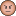# Smart Bluetooth Forum DiscussionsAnonymous
Not applicable

# Hi, i'am trying to use mathematic Functions in my application on the WICED SMART SDK , so i included "math.h" in my c file but i get this error when i use this line double c = sqrt(10);

C:\Users\PC-ASUS\Documents\WICED\WICED-Smart-SDK-2.2.0\WICED-Smart-SDK\Wiced-Smart\spar/../../Apps/wiced_sense/wiced_sense.c:1267: undefined reference to `sqrt'

1 SolutionAnonymous
Not applicable

# Re: Hi, i'am trying to use mathematic Functions in my application on the WICED SMART SDK , so i included "math.h" in my c file but i get this error when i use this line double c = sqrt(10);

i solved the problem was in the conversion from i16_t to double

7 RepliesAnonymous
Not applicable

# Re: Hi, i'am trying to use mathematic Functions in my application on the WICED SMART SDK , so i included "math.h" in my c file but i get this error when i use this line double c = sqrt(10);

i have the same problem all the mathematic functions doesn't work i think there's a problem in the linksAnonymous
Not applicable

# Re: Hi, i'am trying to use mathematic Functions in my application on the WICED SMART SDK , so i included "math.h" in my c file but i get this error when i use this line double c = sqrt(10);

i solved the problem was in the conversion from i16_t to doubleAnonymous
Not applicable

# Re: Hi, i'am trying to use mathematic Functions in my application on the WICED SMART SDK , so i included "math.h" in my c file but i get this error when i use this line double c = sqrt(10);

Hello,

I am trying to use math functions as well and I am also getting errors such as:

undefined reference to `sqrt'

undefined reference to `__aeabi_i2f'

undefined reference to `__aeabi_fmul'

Could you explain how you managed to use math functions included in math.h ?ModeratorModerator# Re: Hi, i'am trying to use mathematic Functions in my application on the WICED SMART SDK , so i included "math.h" in my c file but i get this error when i use this line double c = sqrt(10);Anonymous
Not applicable

# Re: Hi, i'am trying to use mathematic Functions in my application on the WICED SMART SDK , so i included "math.h" in my c file but i get this error when i use this line double c = sqrt(10);

yes i didn't find the way to use that functions even with an external libraries so i used another function that calculate the sqrt only with integers and i multiple the input value (*10,100..) of the function to have the sensibility that i want to get then i devied the output this is the only way i found , the wiced smart sdk do not support floatsAnonymous
Not applicable

# Re: Hi, i'am trying to use mathematic Functions in my application on the WICED SMART SDK , so i included "math.h" in my c file but i get this error when i use this line double c = sqrt(10);

this is the code :

#define NEXT(n, i)  (((n) + (i)/(n)) >> 1)

int abso (int n)

{

if (n<0) return (-n);

else return n ;

}

unsigned int isqrt(int number) {

unsigned int n  = 1;

unsigned int n1 = NEXT(n, number);

while(abso(n1 - n) > 1) {

n  = n1;

n1 = NEXT(n, number);

}

while(n1*n1 > number)

n1--;

return n1;

}Anonymous
Not applicable

# Re: Hi, i'am trying to use mathematic Functions in my application on the WICED SMART SDK , so i included "math.h" in my c file but i get this error when i use this line double c = sqrt(10);